# Detecting Outbreaks and Significant Changes in Signals

This example shows how to determine changes or breakouts in signals via cumulative sums and changepoint detection.

### Detecting Outbreaks via Cumulative Sums

There are many practical applications where you are monitoring data and you want to be alerted as quickly as possible when the underlying process has changed. A very popular technique to achieve this is by means of a cumulative sum (CUSUM) control chart.

To illustrate how CUSUM works, first examine the total reported cases of the 2014 West African Ebola outbreak.

```load WestAfricanEbolaOutbreak2014 plot(WHOreportdate, [TotalCasesGuinea TotalCasesLiberia TotalCasesSierraLeone],'.-') legend('Guinea','Liberia','Sierra Leone'); title('Total suspected, probable and confirmed cases of Ebola virus disease');```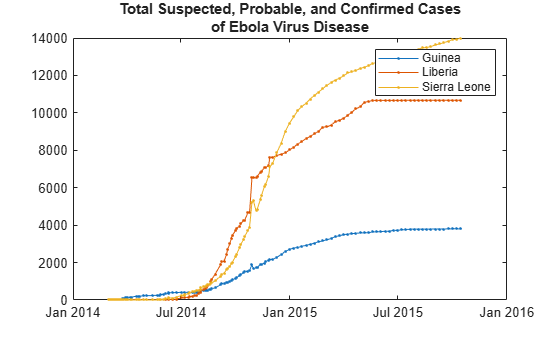If you look at the leading edge of the first outbreak in Guinea, you can see that the first hundred cases were reported around March 25, 2014, and increase significantly after that date. What is interesting to note is that while Liberia also had a few suspected cases in March, the number of cases stayed relatively in control until about thirty days later.

To get a sense of the incoming rate of new patients, plot the relative day-to-day changes in the total number of cases, beginning at the onset on March 25, 2015.

```daysSinceOutbreak = datetime(2014, 3, 24+(0:400)); cases = interp1(WHOreportdate, TotalCasesLiberia, daysSinceOutbreak); dayOverDayCases = diff(cases); plot(dayOverDayCases) title('Rate of new cases (per diem) in Liberia since March 25, 2014'); ylabel('Change in number of reported cases per diem'); xlabel('Number of days since outbreak began');```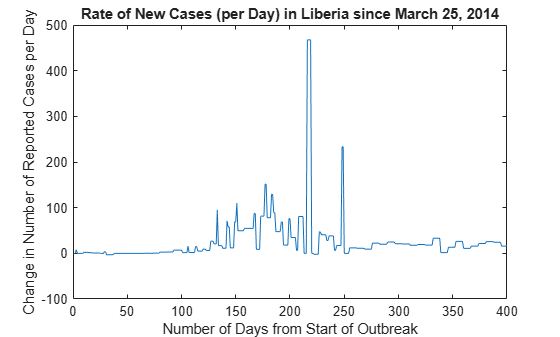If you zoom in on the first hundred days of data, you can see that while there was an initial influx of cases, many of them were ruled out after day 30, where rate of changes dropped below zero temporarily. You also see a significant upward trend between days 95 and 100, where a rate of seven new cases per day was reached.

`xlim([1 101])`Performing a CUSUM test on the input data can be a quick way to determine when an outbreak occurs. CUSUM keeps track of two cumulative sums: an upper sum that detects when the local mean shifts upward, and a lower sum that detects when the mean shifts downward. The integration technique provides CUSUM the ability to ignore a large (transient) spike in the incoming rate but still have sensitivity to steadier small changes in rate.

Calling CUSUM with default arguments will inspect the data of the first twenty-five samples and alarm when it encounters a shift in mean more than five standard deviations from within the initial data.

```cusum(dayOverDayCases(1:101)) legend('Upper sum','Lower sum')```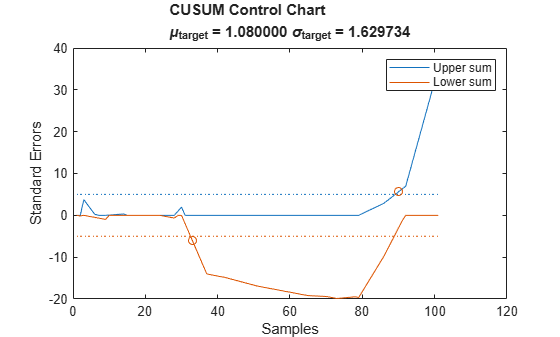Note that CUSUM caught the false reported cases at day 30 (at day 33) and picked up the initial onset of the outbreak starting at day 80 (at day 90). If you compare these results carefully against the previous plot, you can see that CUSUM was able to ignore the spurious uptick at day 29 but still trigger an alarm five days before the large upward trend starting on day 95.

If you adjust CUSUM so that it has a target mean of zero cases/day with a target of plus or minus three cases/day, you can ignore the false alarm at day 30 and pick up the outbreak at day 92:

```climit = 5; mshift = 1; tmean = 0; tdev = 3; cusum(dayOverDayCases(1:100),climit,mshift,tmean,tdev)```### Finding a Significant Change in Variance

Another method of detecting abrupt changes in statistics is through changepoint detection, which partitions a signal into adjacent segments where a statistic (e.g. mean, variance, slope, etc.) is constant within each segment.

The next example analyzes the yearly minimal water level of the Nile river for the years 622 to 1281 AD measured at the Roda gauge near Cairo.

```load nilometer years = 622:1284; plot(years,nileriverminima) title('Yearly Minimum level of Nile River') xlabel('Year') ylabel('Level (m)')```Construction began on a newer more accurate measuring device around 715 AD. Not much is known before this time, but on further examination, you can see that there is considerably less variability after around 722. To find the period of time when the new device became operational, you can search for the best change in the root-mean-square water level after performing element-wise differentiation to remove any slowly varying trends.

```i = findchangepts(diff(nileriverminima),'Statistic','rms'); ax = gca; xp = [years(i) ax.XLim([2 2]) years(i)]; yp = ax.YLim([1 1 2 2]); patch(datenum(xp),yp,[.5 .5 .5],'facealpha',0.1);```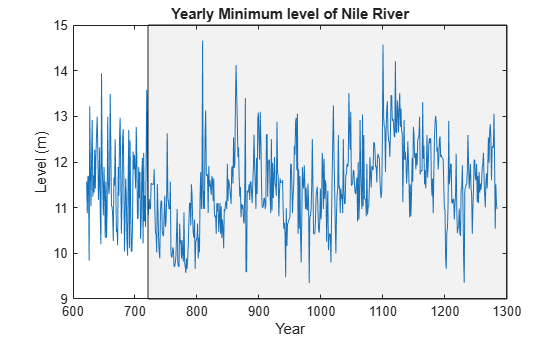While sample-wise differentiation is a simple method to remove trends, there are other more sophisticated methods to examine variance over larger scales. For an example of how to perform changepoint detection via wavelets using this dataset, see Wavelet Changepoint Detection (Wavelet Toolbox).

### Detecting Multiple Changes in an Input Signal

The next example is concerned with a 45 second simulation of a CR-CR 4-speed transmission block, sampled at 1 ms intervals. The simulation data of the car engine RPM and torque are shown below.

```load simcarsig subplot(2,1,2); plot(carTorqueNM); xlabel('Samples'); ylabel('Torque (N m)'); title('Torque'); subplot(2,1,1); plot(carEngineRPM); xlabel('Samples'); ylabel('Speed (RPM)'); title('Engine Speed');```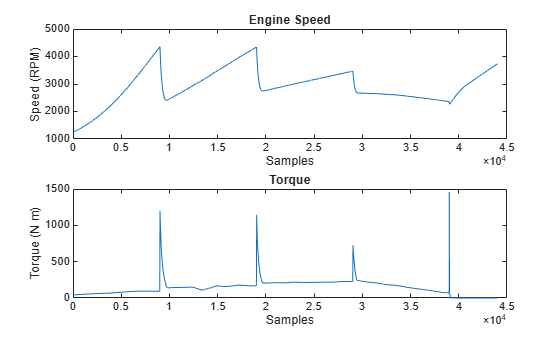Here the car accelerates, changes gears three times, switches to neutral, and then applies the brake.

Since the engine speed can be naturally modeled as a series of linear segments, you can use `findchangepts` to find the samples where the car changes gears.

```figure findchangepts(carEngineRPM,'Statistic','linear','MaxNumChanges',4) xlabel('Samples'); ylabel('Engine speed (RPM)');```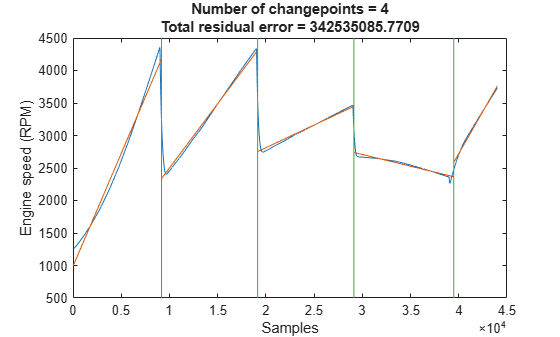Here you can see four changes (between five linear segments) and that they occurred around the 10,000, 20,000, 30,000, and 40,000 sample mark. Zoom into the idle portion of the waveform:

`xlim([18000 22000])`Note that the straight-line fit closely tracks the input waveform, however it can be improved.

### Observing Changes of a Multi-Stage Event Shared Between Signals

To see the improvement, increase the number of changepoints to 20, and observe the changes within the vicinity of the gear change at sample number 19000

```findchangepts(carEngineRPM,'Statistic','linear','MaxNumChanges',20) xlim([18000 22000])```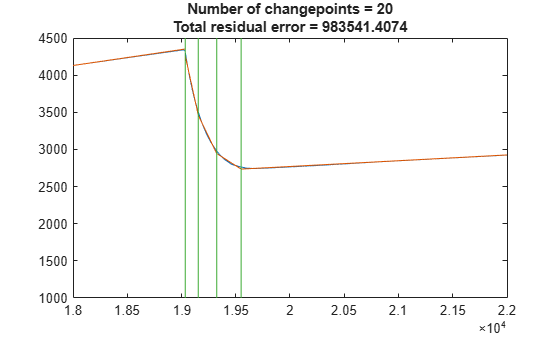Observe that the engine speed started decreasing at sample 19035 and took 510 samples before it settled at sample 19550. Since the sampling interval is 1 ms, this is a ~0.51 s delay and is a typical amount of time after changing gears.

Now look at the changepoints of engine torque within the same region:

```findchangepts(carTorqueNM,'Statistic','Linear','MaxNumChanges',20) xlim([19000 20000])```Observe that the engine torque was fully delivered to the axle at sample 19605, 55 milliseconds after the engine speed finished settling. This time is related to the delay between the intake stroke of the engine and torque production.

To find when the clutch became engaged you can zoom further into the signal.

`xlim([19000 19050])`The clutch was depressed at sample 19011 and took about 30 samples (milliseconds) to become completely disengaged.